# Linear equations and inequalities

## Linear equations

Definition: Linear equation with one variable— an equation of the formwhere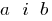is a real number.

If, then the linear equation is also called equation of the first degree.

### Example of solving linear equations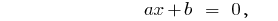then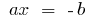Whenis the only root

If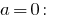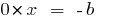(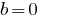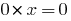— infinite number of roots)

## Linear inequalities

Definition: a Linear inequality in one variableis an inequality of the form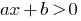where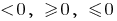is a real number.

If, nerst is also called a linear inequality of the first degree.

### Example of solving linear inequalitythenWhen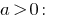When(ifany number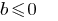— rozvytku no.any number.)

When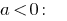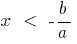Tags:
Chapter:
Versions in other languages:
Share with friends: